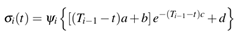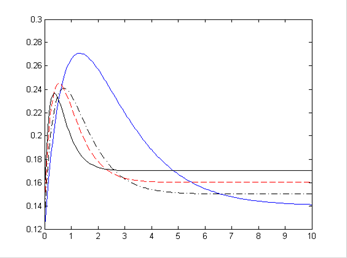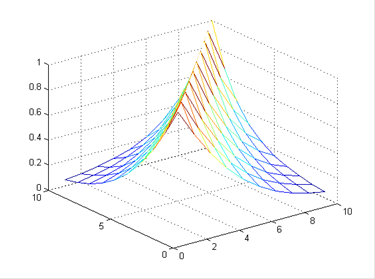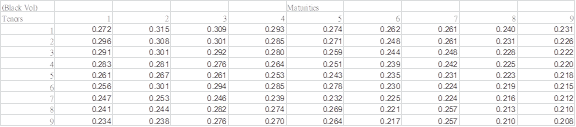Efficient Risk Measure Computation for Bermudan Swaption under LIBOR Market Model

Overview

This software is developed for a general procedure of portfolio risk measure computation, which supports mainly the following two functionalities:

• Calibrate model parameters for some general models
• Given a model and a portfolio of derivatives, compute the price, VaR, CVaR of the portfolio (European-type or Bermudan-type)

We provide the above functionalities in the User Interface as an Excel Form. We perform the computation backend in C++.

Features

• Support calibration of LIBOR Market Model (LMM) under P/Q-measure
• Support pricing / risk measure computation of a portfolio of derivatives, including Bermudan Swaption

We use LSM approach to compute price / risk measure of a general Bermudan Option. Our algorithm on LMM / Bermudan Swaption is based on this paper. In implementing LMM, the user needs to specify the volatility and correlation structure of different LIBOR rates. We only support the following specification of the instantaneous volatility function (as proposed in their paper):The above functional form can result in different shape of volatility function by varying the parameters (a, b, c, d) as illustrated by the following figure. x-axis: time to maturity (T - t); y-axis: instantaneous volatility.For the instantaneous correlation function, we support the S&C 3-parameter model. The model can result in the correlation structure with the following shape:In order to calibrate for the LMM under Q-measure, the user needs to supply a matrix of European swaption volatility in a following form: